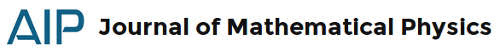### JMP 57, 12: quantum mechanics for the universeI will begin to publish posts in a more continuous way, also recovering some drafts that I'm not able to conclude in 2016. For now I propose you a selection of articles from the Journal of Mathematical Physics, vol.57, issue 12:
Andersson, A. (2016). Electromagnetism in terms of quantum measurements Journal of Mathematical Physics, 57 (12) DOI: 10.1063/1.4972287
We consider the question whether electromagnetism can be derived from the theory of quantum measurements. It turns out that this is possible, both for quantum and classical electromagnetism, if we use more recent innovations such as smearing of observables and simultaneous measurability. In this way, we justify the use of von Neumann-type measurement models for physical processes. We apply the operational quantum measurement theory to gain insight into fundamental aspects of quantum physics. Interactions of von Neumann type make the Heisenberg evolution of observables describable using explicit operator deformations. In this way, one can obtain quantized electromagnetism as a measurement of a system by another. The relevant deformations (Rieffel deformations) have a mathematically well-defined "classical" limit which is indeed classical electromagnetism for our choice of interaction.
Aerts, D., & Sassoli de Bianchi, M. (2016). The extended Bloch representation of quantum mechanics: Explaining superposition, interference, and entanglement Journal of Mathematical Physics, 57 (12) DOI: 10.1063/1.4973356
An extended Bloch representation of quantum mechanics was recently derived to offer a possible (hidden-measurements) solution to the measurement problem. In this article we use this representation to investigate the geometry of superposition and entangled states, explaining interference effects and entanglement correlations in terms of the different orientations a state-vector can take within the generalized Bloch sphere. We also introduce a tensorial determination of the generators of $SU(N)$, which we show to be particularly suitable for the description of multipartite systems, from the viewpoint of the sub-entities. We then use it to show that non-product states admit a general description where sub-entities can remain in well-defined states, even when entangled. This means that the completed version of quantum mechanics provided by the extended Bloch representation, where density operators are also considered to be representative of genuine states (providing a complete description), not only offers a plausible solution to the measurement problem but also to the lesser-known entanglement problem. This is because we no longer need to give up the general physical principle saying that a composite entity exists and therefore is in a well-defined state, if and only if its components also exist and therefore are also in well-defined states.
Hirota, Y. (2016). Geometric quantization of Dirac manifolds Journal of Mathematical Physics, 57 (12) DOI: 10.1063/1.4972779
We extend a prequantization procedure to Dirac manifolds by using singular distributions obtained from 2-cocycles associated with Dirac structures. Given a Dirac manifold $(M, D)$, we describe a prequantization formula in terms of a Lie algebroid connection and show that it is a representation of a Poisson algebra consisting of admissible functions on (M, D) on the space of global sections of a hermitian line bundle over M if and only if the curvature derived from the Lie algebroid connection is represented by a skew-symmetric operation which is naturally defined for $(M, D)$. Moreover, we describe a necessary and sufficient condition for the prequantization formula to be the representation in terms of a Lie algebroid cohomology. We introduce the notion of a polarization for $(M, D)$ and construct a representation of a subalgebra of admissible functions. Lastly, we discuss procedures for quantization in two cases: where M is compact and where $M$ is not compact.
I hope to write something more extensive about the last two papers:
Torres-Vega, G. (2016). A symmetric time-like operator Journal of Mathematical Physics, 57 (12) DOI: 10.1063/1.4973357
We introduce an operator which is the difference between the derivative to the right and to the left, times $i \hbar$, intended to be used in non-relativistic quantum mechanics. This operator is a symmetric, independently of boundary conditions, proposal to a time operator. Some properties of this operator are derived and their eigenstates are found for the free particle system.
Kurianovich, E. (2016). Exact solutions of Friedmann equation Journal of Mathematical Physics, 57 (12) DOI: 10.1063/1.4972836
The cosmological Friedmann equation for the universe filled with a scalar field is reduced to a system of two equations of the first order, one of which is an equation with separable variables. For the second equation, the exact solutions are given in closed form for the potentials as constants and exponents. For the same equation, the exact solutions for a quadratic potential are written in the form of a series in the attractor and spiral areas (inflation stage and the late-time acceleration of the universe, respectively). Also the exact solutions for every arbitrary potential are given in the neighborhood of endpoint and infinity. The existence of all these classical solutions is proven.